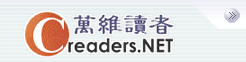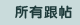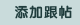设万维读者为首页广告服务技术服务联系我们关于万维简体繁体手机版
 首页 新闻 论坛 博客 视频 分类广告 购物版主：诤友
 五 味 斋 茗香茶语 天下论坛 竞技沙龙 彩虹之约 摄友部落 诗词歌赋 七荤八素 高山流水 海 二 代 教育学术 笑林之声 健康生活 史地人物 军事天地 跨国婚姻 恋恋风尘 灵机一动 股市财经 加国移民 流行前线 新 大 陆 影视娱乐 焦点房谈 我爱我车 美国移民
 万维读者网 > 教育学术 > 帖子
 How to measure return 送交者: jingchen 2019年04月25日16:06:21 于 [教育学术] 发送悄悄话 1. How to measure return?Return is the most fundamental concept in investment. It must be easy to measure. Let’s look at a simple example. Suppose we invest 1000 dollars. After the first year, the investment turns into 2000 dollars. After the second year, the investment turns into 1000 dollars. What is the average annual rate of return? For the first year, the investment doubles. The rate of return is 100%. For the second year, the investment halves. The rate of return is -50%. The average rate of return is therefore 25%. It is simple and clear. This is how standard investment theories, such as portfolio theory and CAPM (Capital Asset Pricing Model), measure investment returns.Some of us may feel uncomfortable with the calculated rate of return. After two years of investment, we don’t make any money. How come the rate of return is 25%? This is the question asked by many people. About three hundred years ago, Daniel Bernoulli considered the same question. He proposed the concept of utility to resolve this issue. In his paper, he introduced the logarithm function to measure utility. Applied to our investment problem, the utility change for the first year is ln(2000)-ln(1000) = ln(2), and the utility change change for the second year is ln(500)-ln(1000) = ln(1/2). The average change of utility is 1/2*(ln(2)+ln(1/2))=0. From utility theory, there is no change in investment value. This is consistent with our intuition. Nowadays, we often use compound return, or geometric return, to measure investment performance. Using compound return, the return of our investment is 0. This is consistent with our intuition. Why we don’t use compound return in the standard theories of investment? When Markowitz developed the portfolio theory, he used arithmetic return and standard deviation to measure investment performance. This measurement was later adopted by Sharpe and others in developing CAPM. These theories have since become the standard in finance and economics. That is why we are stuck with arithmetic return, although it alone, or combined with standard deviation, doesn’t provide precise measurement of investment performance. Is there an investment theory that uses compound return to measure investment performance? The answer is yes. We will discuss that theory in the next section.Behavioral finance 0. Introduction 1. How to measure return 2. How to measure information 3. Theory of investment 4. Theory of asset pricing5. How we process information 6. Common psychological patterns 7. Theory of judgment 8. Investment behaviours and market patterns9. Historical discussion 10. Concluding comments
 0%(0)
 0%(0).ccc{width:675px;display:block;white-space:nowrap;overflow:hidden;float:left;-o-text-overflow: ellipsis;text-overflow:ellipsis;}实用资讯
 一周点击热帖 更多>>
 一周回复热帖
 历史上的今天：回复热帖
 2018: 彭运生谈艺录（208） 2018: 科学家趣闻 2017: 胖子，这提出了重朔中华民族知识产权及 2017: 彭运生谈艺录（16） 2016: 希望中共放下脸面, 知之为知之, 不知为 2016: 定向专业培养排雷老鼠 2015: 认识能力与阶段 - 我们的智力如何逐步 2015: 人生：春天长成的叶子，秋天才能落下来 2014: 朋友在飞机上后边的父母送的，太赞了！ 2014: 在美多元化环境个体更需提